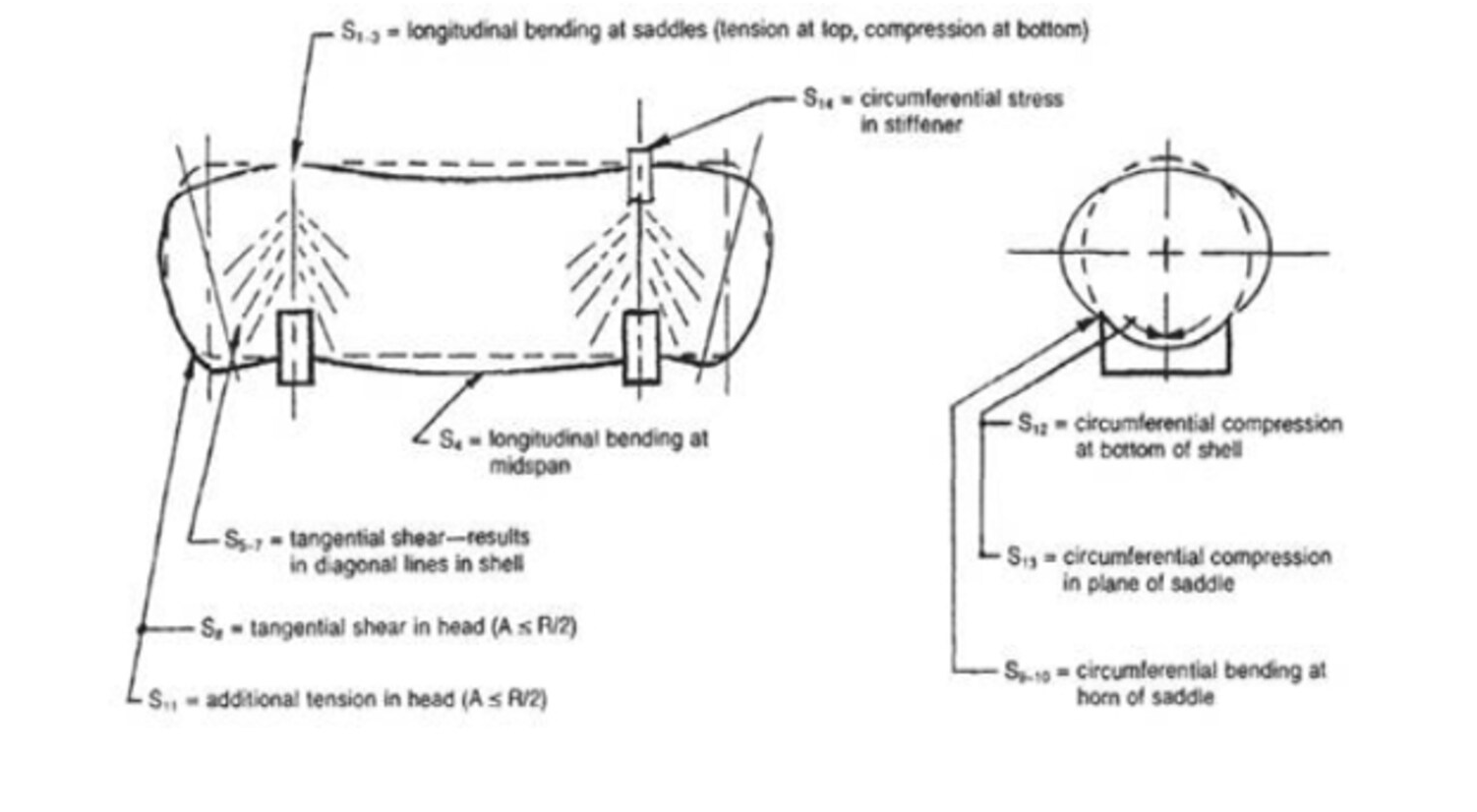Zick analysis is an analzysis method for analysing stresses for the corresponding loads acting on saddles.
In a horizontal vessel there is a chance to act certain types of stress they are
Longitudinal bending stress
Tangential shear stressCircumferential stress

Calculate the stress values of compression, longitudinal, shear, tangential shear, saddle horn bending, and circumferential bending, and then compare those values with the stress limits. If the calculated stress values are less than to stress limits, the design is safe. Otherwise change the dimensions of saddle and then proceed again.
Notations used:
L = Length in inches
R = Radius of Shell in inches
H = depth of Head in inches
Theta = Saddle Contact Angle in degrees
b = Saddle Width in inches
A = Overhang in inches
ts = Corroded Shell Thickness in inches
th = Corroded Head Thickness in inches

Stress limits
Compression Limit Aa = Compression stress geometry factor
Aa = 0.125/(R/ts)
Sh1 limit = compression limit in psi
(compression limit should be taken from material properties of saddle material)
S1 Limit = longitudinal stress limit in psi,
= Sa * E
S2 Limit = shear stress limit in psi
= 0.8 * Sa
S3 Limit = tangential shear limit in psi
= 1.25 * Sa
S4 Limit = saddle horn bending limit in psi
= 1.5 * Sa
S5 Limit = circumferential bending limit in psi
= 0.5 * Sy
Where,
Sa = Allowable shell stress in psi
E = Joint efficiency
R = Radius of shell in inches
ts = Corroded shell thickness in inches